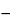Magnesium Oxide
(mag nee' zee um ox' ide).

MgO 40.30
Magnesium oxide[1309-48-4].
DEFINITION
Magnesium Oxide, after ignition, contains NLT 96.0% and NMT 100.5% of MgO.
IDENTIFICATION
A solution in diluted hydrochloric acid meets the requirements.
ASSAY
•  Procedure
Sample:  Ignite 500 mg of Magnesium Oxide to constant weight in a tared platinum crucible.
Analysis:  Dissolve the Sample in 30.0 mL of 1 N sulfuric acid VS, add methyl orange TS, and titrate the excess acid with 1 N sodium hydroxide VS. Perform the blank determination. Calculate the volume, VS, of 1 N sulfuric acid, in mL, consumed by the Sample:
VS = (VBVA) × NNaOH
 VB = = volume of 1 N sodium hydroxide consumed by the blank (mL) VA = = volume of 1 N sodium hydroxide consumed by the Sample (mL) NNaOH = = exact normality of the sodium hydroxide solution
Calculate the volume of 1 N sulfuric acid, VCa, in mL, consumed by calcium, which is present in the portion of Magnesium Oxide taken:
VCa = (W × LCa)/(FCa × 100)
 W = = weight of Magnesium Oxide taken (mg) LCa = = content of calcium as determined in the test for Limit of Calcium (%) FCa = = weight of calcium equivalent to each mL of 1 N sulfuric acid, 20.04 mg
Calculate the percentage of magnesium oxide (MgO) in the portion of Magnesium Oxide taken:
Result = (VSVCa) × (FMgO/W) × 100
 VS = = volume of 1 N sulfuric acid consumed by the sample (mL) VCa = = volume of 1 N sulfuric acid consumed by calcium (mL) FMgO = = weight of magnesium oxide equivalent to each mL of 1 N sulfuric acid, 20.15 mg W = = weight of Magnesium Oxide (mg)
Acceptance criteria:  96.0%–100.5% after ignition
IMPURITIES
•  Free Alkali and Soluble Salts: Boil 2.0 g with 100 mL of water for 5 min in a covered beaker, and filter while hot. Allow to cool. To 50 mL of the cooled filtrate add methyl red TS, and titrate with 0.10 N sulfuric acid. NMT 2.0 mL of the acid is consumed. Evaporate 25 mL of the remaining filtrate to dryness, and dry at 105for 1 h. NMT 10 mg of residue remains (NMT 2.0%).
•  Acid-Insoluble Substances
Sample:  2 g
Analysis:  Mix the Sample with 75 mL of water, add hydrochloric acid in small portions, with agitation, until no more dissolves, and boil for 5 min. If an insoluble residue remains, filter, wash well with water until the last washing is free from chloride, and ignite.
Acceptance criteria:  The weight of the ignited residue is NMT 2 mg (NMT 0.1%).
•  Limit of Calcium
[Note—A commercially available atomic absorption standard solution for calcium may be used where preparation of a calcium standard stock solution is described below. Concentrations of the Standard solutions and the Sample solution may be modified to fit the linear or working range of the instrument. ]
Dilute hydrochloric acid:  Dilute 100 mL of hydrochloric acid with water to 1000 mL.
Lanthanum solution:  To 58.65 g of lanthanum oxide add 400 mL of water, and add, gradually with stirring, 250 mL of hydrochloric acid. Stir until dissolved, and dilute with water to 1000 mL.
Standard solutions:  Transfer 249.7 mg of calcium carbonate, previously dried at 300for 3 h and cooled in a desiccator for 2 h, to a 100-mL volumetric flask. Dissolve in a minimum amount of hydrochloric acid, and dilute with water to volume. Transfer 1.0, 5.0, 10.0, and 15.0 mL of this stock solution to separate 1000-mL volumetric flasks, each containing 20 mL of the Lanthanum solution and 40 mL of Dilute hydrochloric acid, and dilute with water to volume. These Standard solutions contain 1.0, 5.0, 10.0, and 15.0 µg/mL of calcium, respectively.
Sample solution:  Transfer 250 mg of Magnesium Oxide, freshly ignited, to a beaker. Add 30 mL of Dilute hydrochloric acid, and stir until dissolved, heating if necessary. Transfer the solution so obtained to a 200-mL volumetric flask containing 4 mL of Lanthanum solution, and dilute with water to volume.
Blank solution:  Transfer 4 mL of the Lanthanum solution and 10 mL of Dilute hydrochloric acid to a 200-mL volumetric flask, and dilute with water to volume.
Instrumental conditions
Mode:  Atomic absorption spectrophotometry
Analytical wavelength:  Calcium emission line at 422.7 nm
Lamp:  Calcium hollow-cathode
Flame:  Nitrous oxide–acetylene
Analysis
Samples:  Standard solutions, Sample solution, and Blank solution
Using the calibration graph, determine the concentration, C, in µg/mL, of calcium in the Sample solution.
Calculate the percentage of calcium in the portion of Magnesium Oxide taken:
Result = (V/W × C × F) × 100
 V = = volume of the Sample solution (mL) W = = weight of Magnesium Oxide (mg) C = = concentration of calcium in the Sample solution (µg/mL) F = = conversion from µg/mL to mg/mL, 0.001
Acceptance criteria:  NMT 1.1%
Test preparation:  Dissolve 2.0 g in 35 mL of 3 N hydrochloric acid, and evaporate the solution on a steam bath to dryness. Toward the end of the evaporation, stir frequently to disintegrate the residue so that finally a dry powder is obtained. Dissolve the residue in 20 mL of water, and evaporate to dryness in the same manner as before. Redissolve the residue in 20 mL of water, filter if necessary, and dilute with water to 40 mL. To 20 mL, add water to make 25 mL.
Acceptance criteria:  NMT 20 ppm
Test preparation:  Boil 40 mg with 5 mL of 2 N nitric acid for 1 min. Cool, dilute with water to 50 mL, and mix. Dilute 25 mL of this solution with water to 45 mL, and add 2 mL of hydrochloric acid.
Acceptance criteria:  NMT 0.05%
SPECIFIC TESTS
Transfer 500 mg to a tared platinum crucible, and ignite at 800 ± 25to constant weight: it loses NMT 10.0% of its weight.
Using the procedure specified in the chapter, determine the bulk density of Magnesium Oxide.